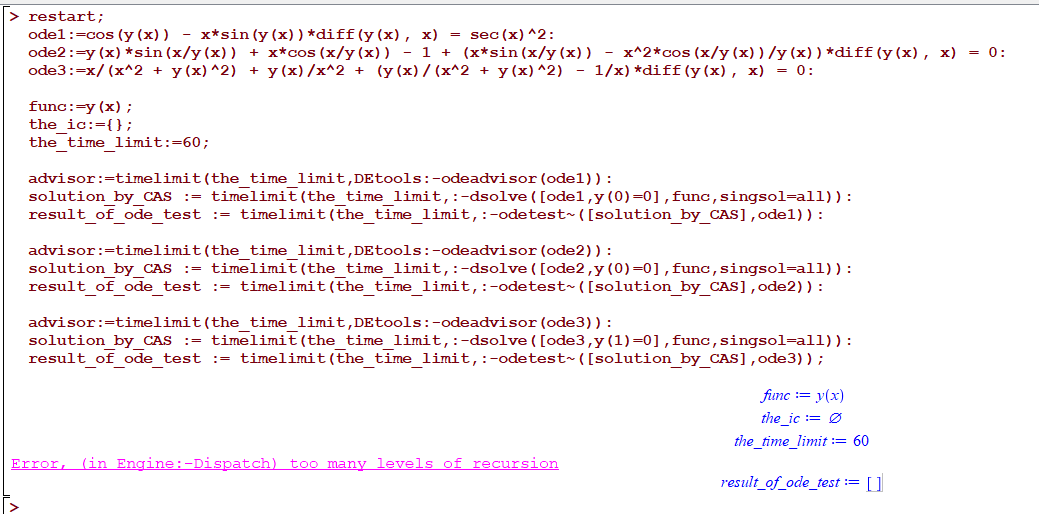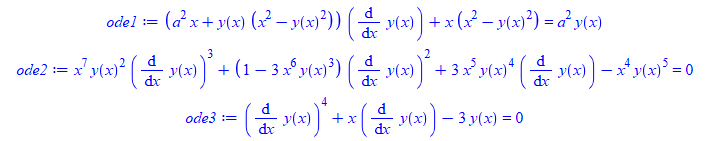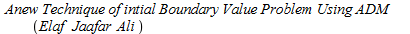## random error Error, (in Engine:-Dispatch) too many...

it took a while to track this. When I run these 3 ode's in this sequence, Maple gives

Error, (in Engine:-Dispatch) too many levels of recursion

and it has to be this sequence.  I also found I might have to run the code 2 or 3 times to get this error if it does not show first time.

Will show a movie below also.

Here is the code

```restart;
ode1:=cos(y(x)) - x*sin(y(x))*diff(y(x), x) = sec(x)^2:
ode2:=y(x)*sin(x/y(x)) + x*cos(x/y(x)) - 1 + (x*sin(x/y(x)) - x^2*cos(x/y(x))/y(x))*diff(y(x), x) = 0:
ode3:=x/(x^2 + y(x)^2) + y(x)/x^2 + (y(x)/(x^2 + y(x)^2) - 1/x)*diff(y(x), x) = 0:

func:=y(x);
the_ic:={};
the_time_limit:=60;

solution_by_CAS := timelimit(the_time_limit,:-dsolve([ode1,y(0)=0],func,singsol=all)):
result_of_ode_test := timelimit(the_time_limit,:-odetest~([solution_by_CAS],ode1)):

solution_by_CAS := timelimit(the_time_limit,:-dsolve([ode2,y(0)=0],func,singsol=all)):
result_of_ode_test := timelimit(the_time_limit,:-odetest~([solution_by_CAS],ode2)):

solution_by_CAS := timelimit(the_time_limit,:-dsolve([ode3,y(1)=0],func,singsol=all)):
result_of_ode_test := timelimit(the_time_limit,:-odetest~([solution_by_CAS],ode3));
```And here is a movie (this time it happend on second run) sometimes it happens on first run. so it is random.Maple 2020.1

Why does it happen and is there a work around?

## few errors using dsolve and and odetest...

Noticed few errors generated on some ode's. I do not know if these are expected or known, I thought may be someone might want to check them.

```restart;
ode1:=(a^2*x+y(x)*(x^2-y(x)^2))*diff(y(x),x)+x*(x^2-y(x)^2) = a^2*y(x);
ode2:=x^7*y(x)^2*diff(y(x),x)^3+(1-3*x^6*y(x)^3)*diff(y(x),x)^2+3*x^5*y(x)^4*diff(y(x),x)-x^4*y(x)^5 = 0;
ode3:=diff(y(x),x)^4+x*diff(y(x),x)-3*y(x) = 0;
````sol:=timelimit(60,:-dsolve(ode1,y(x))):`

Error, (in dsolve) numeric exception: division by zero

```sol:=timelimit(60,:-dsolve(ode2,y(x))):
timelimit(60,:-odetest([sol],ode2))
```

Error, (in IntegrationTools:-Indefinite:-AlgebraicFunction) argument is not an algebraic

```sol:=timelimit(60,:-dsolve(ode3,y(x))):
timelimit(60,:-odetest(sol,ode3));
timelimit(60,:-odetest(sol,ode3))
```

Error, (in odetest) received not an ODE w.r.t x(_T)
Error, (in odetest) received not an ODE w.r.t y(_T)

This last one is only due to how the solution is given. So I do not think it is a real problem.

Maple 2020.1, Physics 708

windows 10

Maple Worksheet - Error
Failed to load the worksheet /maplenet/convert/check.mw .

## How do I solved PDE using non classical numerical ...

Dear All.

I hope we are all staying safe.

Please I want to solve Sine Gordon Equation using a numerical method I constructed (non-classical), I need to compare the result of the method with the exact solution to generate the errors. However, since the exact solution has two variables, x, and t, I really don't know how to accommodate the two in my coding.

Can someone be of help in this regard?

Thank you and kind regards

## Why inequal plot like this?...

Hi there,

Can someone explain to me why is it happening? If smaller equal than coloured well, if smaller then just the equal line appears. Why?

```with(plots);
dm1 := .23; pm12 := .7; dm12 := .23;
inequal(pm1*(dm12+am21)-pm12*(dm1+am21) <= 0, pm1 = 0 .. 1, am21 = 0 .. 1, color = "Nautical 1");

````inequal(pm1*(dm12+am21)-pm12*(dm1+am21) < 0, pm1 = 0 .. 1, am21 = 0 .. 1, color = "Nautical 1")`Thank you!

## Ellipse Equation ( rotation)...

Hi ,

i want to find simple equation of rotate Ellipse  ( El1,EL2,El3 in the  worksheet), with calssic formulaIdeas? Thanks

QuestionConiqueRotation.mw

## Find the focus of an ellipse with a tangential gen...

Etude d'un cas particulier a := 5: b := 7: k := 9: A := [a, 0]: B := [0, b]: #A et B fixes P := [t, 0]: Q := [0, k/t]:#P et Q 2 points mobiles cir := -a*x-b*y+x^2+y^2 = 0: sol := solve(subs(y = 5, cir), x): cen := [solve(diff(cir, x)), solve(diff(cir, y))]: x0 := sol: y0 := 5: M := [x0, y0]: R := sqrt(cen^2+cen^2): beta := arctan(diff(solve(EQ(M, cen), y), x)): Recherche des valeurs de t pour que les 2 droites soient perpendiculaires eq := t^2*(y0-b)+t*(a*b-a*y0+b*x0-k)-x0*(a*b-k) = 0; sol := solve(eq, t); t := sol; tp := sol; P1 := [t, 0]; Q1 := [0, k/t]; PQ1 := simplify(x*(-a*b+b*t+k)+y*t*(t-a)-t*(-a*b+b*t+k)) = 0:#1ere tangente PQ2 := simplify(x*(-a*b+b*tp+k)+y*tp*(tp-a)-tp*(-a*b+b*tp+k)) = 0:#2ième tangente P2 := [tp, 0]; Q2 := [0, k/tp]; CIR := implicitplot(cir, x = -4 .. 8, y = -4 .. 12, color = red); Fig := proc (alpha) local Dr1, DR1, Dr2, DR2, N, u0, v0, Po, t, tp, sol; global a, b, k, cen, R; u0 := cen+R*cos(alpha); v0 := cen+R*sin(alpha); N := [u0, v0]; sol := solve(t^2*(v0-b)+t*(b*u0-a*v0+a*b-k)-u0*(a*b-k) = 0, t); t := sol; tp := sol; Dr1 := simplify(x*(-a*b+b*t+k)+y*t*(t-a)-t*(-a*b+b*t+k)) = 0; DR1 := implicitplot(Dr1, x = -4 .. 8, y = -4 .. 12, color = brown); Dr2 := simplify(x*(-a*b+b*tp+k)+y*tp*(tp-a)-tp*(-a*b+b*tp+k)) = 0; DR2 := implicitplot(Dr2, x = -4 .. 8, y = -4 .. 12, color = pink); Po := pointplot([N[]], symbol = solidcircle, color = [black], symbolsize = 8); display([Po, DR1, DR2]) end proc; DrPQ1 := implicitplot(PQ1, x = -4 .. 22, y = -4 .. 12, color = blue); DrPQ2 := implicitplot(PQ2, x = -4 .. 22, y = -4 .. 12, color = blue); Points := pointplot([A[], B[], M[], P1[], P2[], Q1[], Q2[], cen[]], symbol = solidcircle, color = [green], symbolsize = 10); T := plots:-textplot([[A[], "A"], [B[], "B"], [M[], "M"], [P1[], "P1"], [P2[], "P2"], [Q1[], "Q1"], [Q2[], "Q2"], [cen[], "cen"]], font = [times, 10], align = {below, left}); n := 19; display([seq(Fig(2*i*Pi/n), i = 0 .. n), Fig(beta), CIR, DrPQ1, DrPQ2, Points, T], scaling = constrained, size = [500, 500]); I would find out the focus of the ellipse. Thank you.

## Homotopy Analysis Method, NOPH package and solutio...

Hello, dear forum users!

Does anyone use the method of homotopy analysis (HAM) and the NOPH package in their work. (moderator: link)It seems to me that only HAM can help.

There are no developments, as I do not own the NOPH package.

## How to make  "with(combinat)"work in Maple Linux...

When I type "with(combinat)" in Maple Linux, I got the following erros message:

"Error, invalid input: with expects its 1st argument, pname, to be of type {`module`, package}, but received combinat"

So how to make it work?

Many thanks.

## `Anew Technique of intial Boundary Value Problem U...

Hello! hope you all be fine. i m running a code for `Anew Technique of intial Boundary Value Problem Using ADM `(Elaf*Jaafar*Ali)but my code is for positive integer and i want to run it for fractional can anyone help me please code file is attached

## How to simply a equation like textbook, Maple use ...

The pdf shows the derivation procedure of Image from 1.2.1 to 1.2.5. However, when we obtain the (7) in pdf, which is the

expansion of 1.2.4, we hope to see the hamiltonian operator in the output.  We obtain the (10) in pdf (Maple). Actually, it is the expansion of 1.2.5. However, it is too cockamamie compared with that (1.2.5) in the textbook.

Hence, I hope to show the hamiltonian operator in the (10) in PDF. I hope to see the simplest type of equation.

How could I do? Appreciate the kind help from anyone!

The Maple code is also uploaded.(1)(2)(3)(4)(5)(6)(7)(8)(9)(10)20200619.pdf## Compiler package setup...

Error, (in Compiler:-Setup) unable to write to initialization file, C:\Program Files\Maple 2020\bin.X86_64_WINDOWS/launch.ini, possibly due to insufficient permissions
NULL;

when choose bat file

but i see sufficient permission

any w should i s

choose a particular compiler or even visual studio i have in my system

where can i find if anything else

## Error importing a big Excel data file xlsx...

Hello,

I try import a big Excel file with the first row as column labels for performing statistical etc.

I use this command :

data := Import(FileTools:-JoinPath(["d:", "directory", "subset2.xlsx"], platform = "windows"))

i need a dataframe for performing statistical with column label as first row, but i have this error :

Error, (in Import) invalid input: MatrixToDataFrame uses a 3rd argument, columns, which is missing

I can not understand what is this error...so, what i can do ?

Thanks, regards

## wrong result working with differential forms?...

This is my notebook

 >>>M >M >(1)
 M >(2)
 M >
 >I don't understand why computing the wedge product of two differential forms gives me an output which prints the product of both. Why  don't it just return d rho ^ dt?

## How to plot a function at a point 0....

Dear Maple Users @acer @Carl Love @Kitonum @Preben Alsholm ,

Greetings.

How to plot a function "Am" for various values of "kt"(eg: kt=-1..1) at a point x=0.

Am vs kt.

The code has provided below.

ktplot.mw

## implicitplot3d+contourplot...

Hi experts

with(plots):
#epsilon:=5*Pi/2:
gammaa:=0.4:
gammao:=0.1:
gammab:=0.002:
omegab:=100:
X:=60:
Omega:=6*Pi:
g:=10:
sigmag:=5*gammaa:
a:=1/(sigmag*sqrt(2*Pi)):

#deltao:=1:
D7:=evalf(a*int(g*Omega*(gammao/2-I*Delta)*exp(-0.5*((Delta-deltao)/sigmag)^2)/(gammao^2/4+Delta^2),Delta=-infinity..infinity)):
D8:=evalf(0.5*a*int(g^2*gammao*exp(-0.5*((Delta-deltao)/sigmag)^2)/(gammao^2/4+Delta^2), Delta=-infinity..infinity)):
D9:=evalf(a*int(g^2*Delta*exp(-0.5*((Delta-deltao)/sigmag)^2)/(gammao^2/4+Delta^2), Delta=-infinity..infinity)):
b1 := (deltao-2*X^2*omegab*Y/(gammab^2+omegab^2)-D9)^2:

f:=Y*((gammaa+D8)^2+(b1))=Im(D7)^2+(epsilon-Re(D7))^2:
#P1:=implicitplot(f,deltao=-70..50,Y=0..1,numpoints=100,axes=boxed,thickness=2,color=black,font=[1,1,20],tickmarks=[4, 3],linestyle=1);

implicitplot3d(f,Y = 0 .. 1,deltao=-40..40,epsilon=0..0.4,numpoints=100,labels=[Y,deltao,E],tickmarks=[3,3,3], style = surface, color = "Niagara Azure");

The above code to plot the implicitplot3d ,

first the figures are not smooth

second thing is how to make contourplot at epsilon= 5*Pi/2.